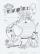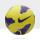# Target

Peter, Martin and Jirka were fire in a special target, which had only three fields with values of 12, 18 and 30 points. All boys were firing with the same number of arrows and all the arrows hit the target, and the results of every two boys differed in one point.

Peter's average score was two points better than Martin's and Martin was one point better than Jirka's average.

Find how many arrows each of the boys fired.

Result

n =  6

#### Solution:

Peter: 30 30 30 30 30 30; AVG = 30
Martin: 30 30 30 30 30 18; AVG = 28
Jirka: 30 30 30 30 30 12; AVG = 27

Program in PHP:
```\$x = array(12,18,30);
\$ch = array();
for(\$n=1;\$n<1000;\$n++)
{
foreach(\$x AS \$xx)
{
\$ch[\$n] = \$xx;

foreach(\$x AS \$yy)
{
\$ch[\$n] = \$yy;
foreach(\$x AS \$zz)
{
\$ch[\$n] = \$zz;

\$s0 = array_sum(\$ch);
\$s1 = array_sum(\$ch);
\$s2 = array_sum(\$ch);

if(\$s0/\$n != 2+\$s1/\$n || \$s1/\$n != 1 + \$s2/\$n)
{
continue;
}

echo(\$n);
print_r(\$ch);

}
}
}

}
```

Leave us a comment of example and its solution (i.e. if it is still somewhat unclear...):Be the first to comment!#### To solve this verbal math problem are needed these knowledge from mathematics:

Looking for help with calculating arithmetic mean? Looking for a statistical calculator? Do you have a linear equation or system of equations and looking for its solution? Or do you have quadratic equation?

## Next similar examples:

1. NutsPeter has 49 nuts. Walnuts have 3 more than hazelnuts and hazelnut 2 over almonds. Determine the number of wallnuts, almonds and hazelnuts.
2. Men, women and childrenOn the trip went men, women and children in the ratio 2:3:5 by bus. Children pay 60 crowns and adults 150. How many women were on the bus when a bus was paid 4,200 crowns?
3. Exam averageAverage of marks that have on the certificates students of 8.A class in mathematics is exactly 2.45. If we did not add 1 and 3 of sutdents Michael and Alena, who arrived a month ago, it would average exactly 2.5. Determine how many students have class 8.
4. Three workshopsThere are 2743 people working in three workshops. In the second workshop works 140 people more than in the first and in third works 4.2 times more than the second one. How many people work in each workshop?
5. 75th percentile (quartille Q3)Find 75th percentile for 30,42,42,46,46,46,50,50,54
6. Median and modusRadka made 50 throws with a dice. The table saw fit individual dice's wall frequency: Wall Number: 1 2 3 4 5 6 frequency: 8 7 5 11 6 13 Calculate the modus and median of the wall numbers that Radka fell.
7. Linsys2Solve two equations with two unknowns: 400x+120y=147.2 350x+200y=144
8. ChildrenThe group has 42 children. There are 4 more boys than girls. How many boys and girls are in the group?
9. LegsCancer has 5 pairs of legs. The insect has 6 legs. 60 animals have a total of 500 legs. How much more are cancers than insects?
10. Ball gameRichard, Denis and Denise together scored 932 goals. Denis scored 4 goals over Denise but Denis scored 24 goals less than Richard. Determine the number of goals for each player.
11. Elimination methodSolve system of linear equations by elimination method: 5/2x + 3/5y= 4/15 1/2x + 2/5y= 2/15
12. Three unknownsSolve the system of linear equations with three unknowns: A + B + C = 14 B - A - C = 4 2A - B + C = 0
13. AverageIf the average(arithmetic mean) of three numbers x,y,z is 50. What is the average of there numbers (3x +10), (3y +10), (3z+10) ?
14. Theorem proveWe want to prove the sentence: If the natural number n is divisible by six, then n is divisible by three. From what assumption we started?
15. TeamThe football team has 11 players. Their average age is 20 years. During the match due to injury, one player withdraws and the average age of the team is 18. How old was injured player?
16. Average ageThe company of five people has an average age of 46 years. The average age of the first four is 43 years. How many years has the fifth member of this company?
17. Difference of two numberThe difference of two numbers is 20. They are positive integers greater than zero. The first number raised to one-half equals the second number. Determine the two numbers.